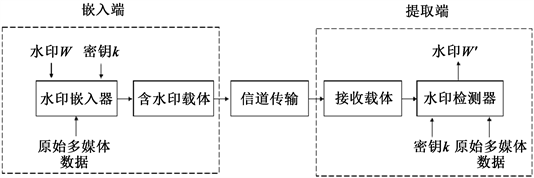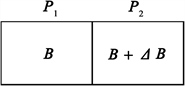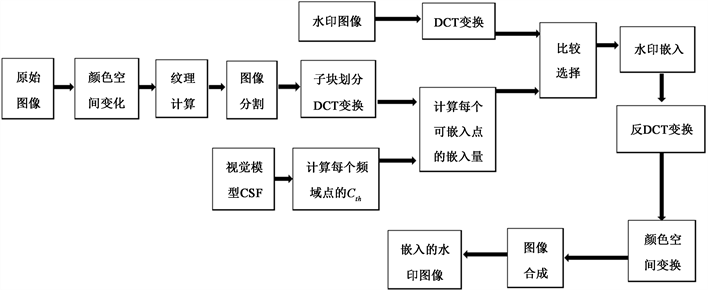#### 期刊菜单

Design and Implementation of Image Watermarking System Based on Visual Features

Abstract: With the development of the Internet, digital works such as images and audio have proliferated, the infringement of digital works is becoming increasingly serious. As digital works are easy to be copied and spread, the problem of infringement of digital works is more and more serious. Digital watermarking is a very effective means of copyright protection. Digital watermarking technology can protect copyright effectively. Digital watermarking technology can mask the human visual system (HVS) by using the contrast and characteristics of visual feature-sensitive texture images through analyzing the characteristics of human vision and the visual masking characteristics of important fields. Finally, the watermark information is adaptively included in the DFT domain name of the important domain. The results show that this algorithm is an effective image watermarking algorithm, which can effectively solve the balance between watermark capacity, visual transparency and robustness in the process of watermark embedding.

1. 课题背景和研究意义

2. 数字水印技术Figure 1. Digital watermarking system framework

3. 人类视觉特征Figure 2. Luminance perception characteristics of human eyes

1) 由于 $\Delta {B}_{\mathrm{min}}$ 不是无限小量，所以两者亮度差达到 $\Delta {B}_{\mathrm{min}}$ (可见度阈值)时，人眼才能感觉P1和P2两个图片是具有亮度差别的。由于 $\Delta {B}_{\mathrm{min}}$ 不是无限小量。

2) 可察觉的最小亮度差 $\Delta {B}_{\mathrm{min}}$ 因背景亮度B不同而不同。但在一个均匀亮度背景下， $\Delta {B}_{\mathrm{min}}/B$ 是相同的，并等于一个常数 $\xi \ast \xi =\Delta {B}_{\mathrm{min}}/B$ 称为相对对比灵敏度阈。随着环境的不同， $\xi$ 的值在0.005到0.020范围内变化。当背景亮度很高时， $\xi$ 的值甚至可以增大至0.050。

1) 亮度感知的CSF

${\text{CFS}}_{\text{L}}\left({f}_{\theta }\right)=a\cdot {f}_{\theta }\cdot \mathrm{exp}\left(-b\cdot {f}_{\theta }\right)\left[1+c×\mathrm{exp}\left(b×{f}_{\theta }\right)\right]$ (1)

$a,b,c$ 为参数， ${f}_{\theta }$ 为对象光栅感知时的角频率

$a=\frac{540\cdot {\left(1+0.7/L\right)}^{-0.2}}{1+\frac{12}{\omega \cdot {\left(1+{f}_{\theta }/3\right)}^{2}}},\text{\hspace{0.17em}}b=0.3\cdot {\left(1+100/L\right)}^{0.15},\text{\hspace{0.17em}}c=0.06$ (2)

L表示对象光栅的平均亮度； $\omega$ 表示感知对象光栅时刺激瞳孔的大小。

2) 对立色感知的CSF

${\text{CFS}}_{\text{rg}}\left({f}_{\theta }\right)=a\cdot {\mathrm{e}}^{b\left({f}_{\theta }\right)c}$

$a=1,\text{\hspace{0.17em}}b=-0.152,\text{\hspace{0.17em}}c=0.893$ (3)

${\text{CFS}}_{\text{by}}\left({f}_{\theta }\right)=a\cdot {\mathrm{e}}^{b\left({f}_{\theta }\right)c}$

$a=1,\text{\hspace{0.17em}}b=-0.2041,\text{\hspace{0.17em}}c=0.9$ (4)

CFSrg和CFSby描述为人眼对红绿和蓝黄对立色光栅时的彩色对比敏感度阈值 。

4. 视觉特征在图像水印中的应用及水印算法设计

${f}_{\theta }=\frac{N}{\theta }$ (5)

${f}_{\theta }=\frac{\sqrt{{f}_{x}^{2}+{f}_{y}^{2}}}{\theta }$ (6)Figure 3. Flow chart of watermark embedding scheme

${I}_{i}={I}_{i1}+{I}_{i2},\text{\hspace{0.17em}}\text{\hspace{0.17em}}i=L,rg,by$ (7)

${W}_{iTh}={C}_{iTh}\left({f}_{\theta }\right)×{I}_{i1},\text{\hspace{0.17em}}\text{\hspace{0.17em}}i=L,rg,by$ (8)

$\begin{array}{l}\frac{abs\left(K×{W}_{i}-{W}_{iTh}\right)}{{W}_{Th}}<5%,\\ {W}_{is}=K×{W}_{i},\text{\hspace{0.17em}}\text{\hspace{0.17em}}i=L,rg,by,\\ \text{else}\text{\hspace{0.17em}}\text{ }\text{ }{W}_{is}=0\end{array}$ (9)

5. 结论

  周凯. 视觉特征引导的彩色图像水印算法研究[D]: [硕士学位论文]. 济南: 山东师范大学, 2021.  王俊. 基于鲁棒视觉属性的量化水印方法研究[D]: [硕士学位论文]. 济南: 山东师范大学, 2020.  申静. 基于视觉特性和纹理特征的图像水印算法研究[J]. 液晶与显示, 2018, 33(6): 511-519.  赵博. 基于数字水印的图像内容认证研究[D]: [博士学位论文]. 长春: 吉林大学, 2018.  王玮, 石胜辉. 人类视觉特性的数字水印新方案[J]. 华侨大学学报(自然科学版), 2016, 37(3): 304-307.  王晶. 基于内容特征的数字图像水印方法研究[D]: [硕士学位论文]. 大连: 辽宁师范大学, 2011.  任璐. 基于视觉注意机制的数字水印技术研究[D]: [硕士学位论文]. 西安: 西安电子科技大学, 2011.